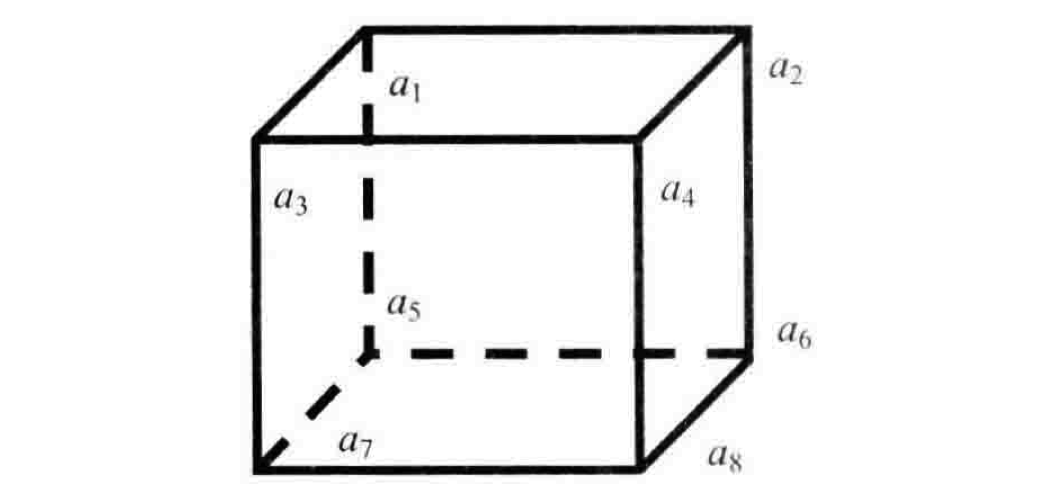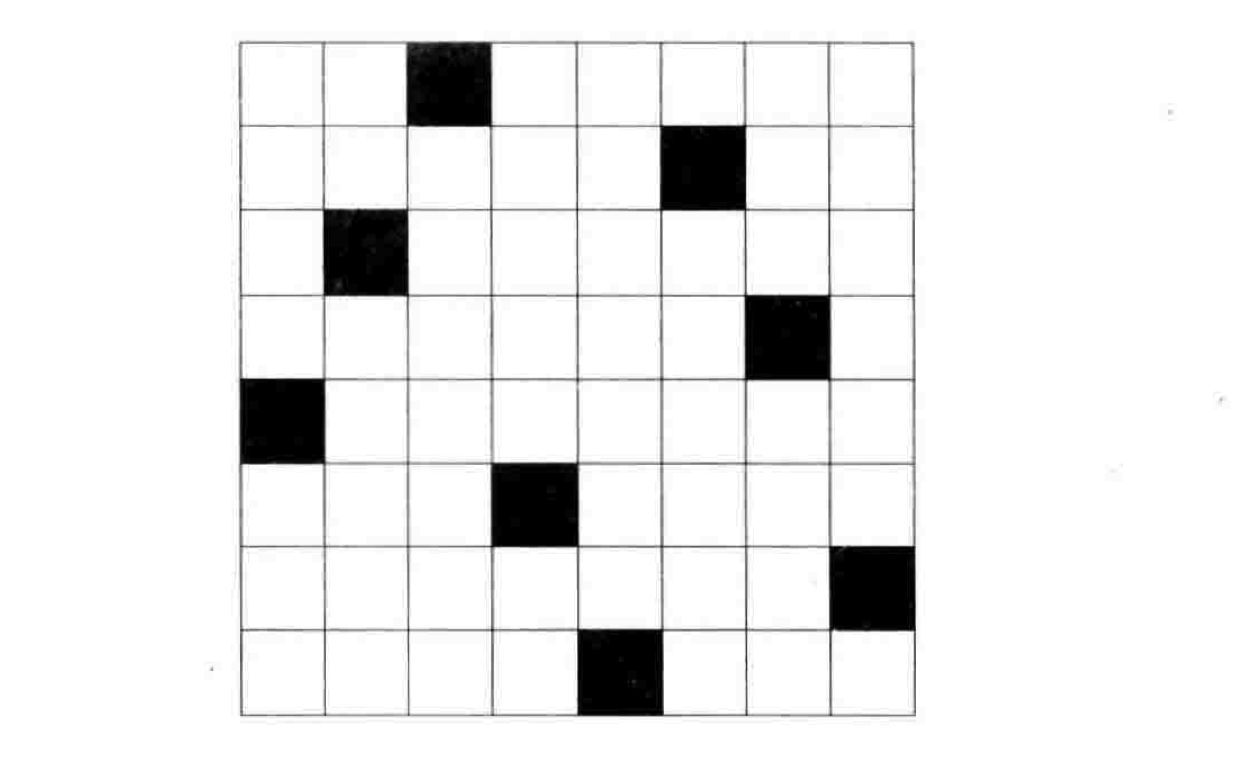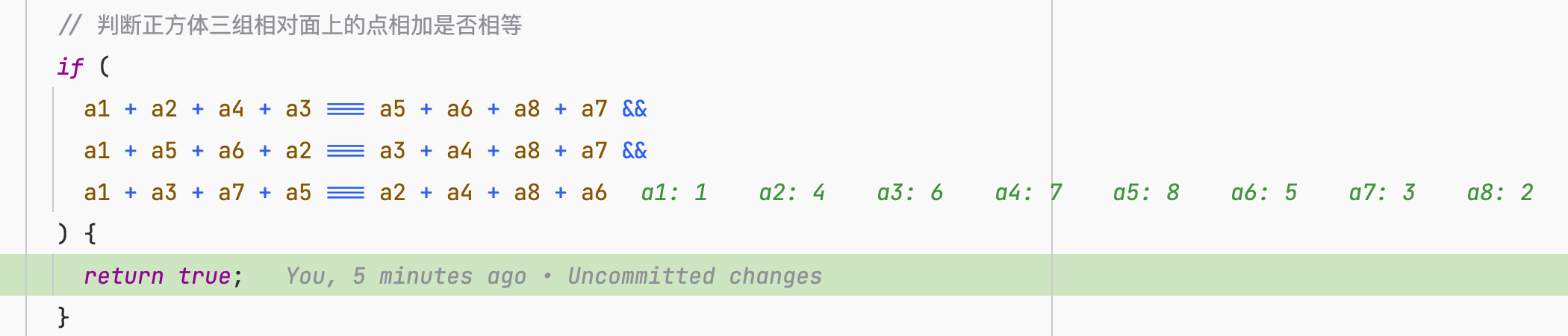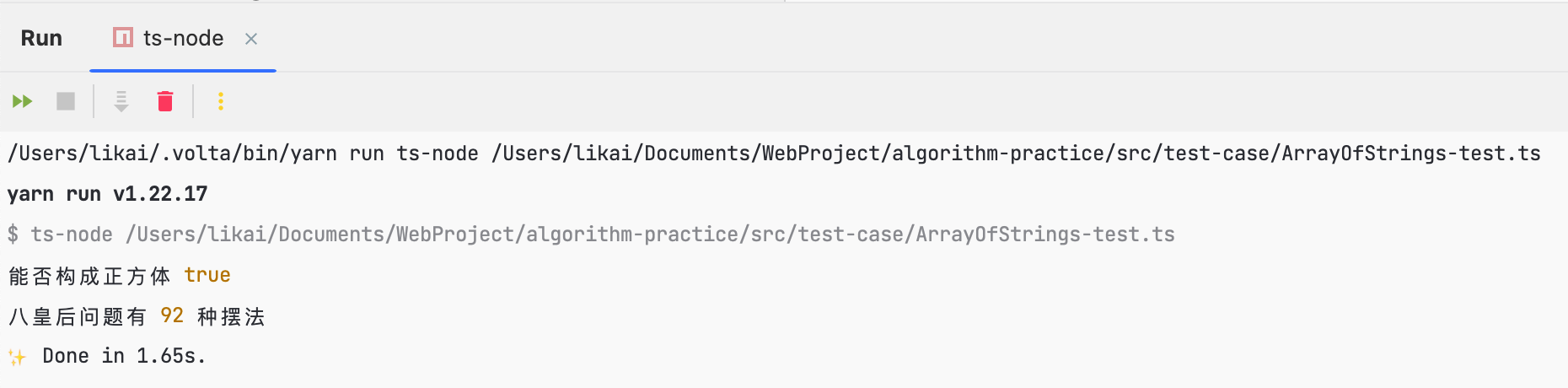• 累计撰写 164 篇文章
• 累计创建 25 个标签
• 累计收到 208 条评论

### 目 录CONTENT# 全排列的应用：正方体的组成与八皇后2023-06-26 / 0 评论 / 17 点赞 / 1,107 阅读 / 2,988 字

## 正方体的组成### 实现思路

• a1, a2, a4, a3 | a5, a6, a8, a7
• a1, a5, a6, a2 | a3, a4, a8, a7
• a1, a3, a7, a5 | a2, a4, a8, a6

8个数字分别放到8个顶点上，所有数字都有可能放入任意一个顶点。换言之就是求这8个数字的所有排列，我的另一篇文章实现字符串的排列算法详细讲解了这个算法的实现思路，此处不过多赘述。

• 求出给定数组中8个数字的所有排列
• 遍历所有排列，将每个排列中的元素映射到变量中(a1, a2, …, a8)
• 判断8个点组成的三组相对面的顶点和是否相等

### 实现代码

  /**
* 能否构成正方体
* @param nums
*/
public isCubePossible(nums: Array<number>): boolean {
if (nums.length !== 8) {
return false;
}
// 获取8个点的所有排列
const list = this.permute(nums.join(""));
for (let i = 0; i < list.length; i++) {
// 将当前组合中的点转为number类型放入item
const item: Array<number> = [];
for (let j = 0; j < list[i].length; j++) {
item.push(+list[i][j]);
}
// 从当前组合中获取正方体的8个点
const [a1, a2, a3, a4, a5, a6, a7, a8] = item;
// 判断正方体三组相对面上的点相加是否相等
if (
a1 + a2 + a4 + a3 === a5 + a6 + a8 + a7 &&
a1 + a5 + a6 + a2 === a3 + a4 + a8 + a7 &&
a1 + a3 + a7 + a5 === a2 + a4 + a8 + a6
) {
return true;
}
}
return false;
}



## 八皇后问题### 实现思路

• 定义皇后数组，分别用0～7对这个数组进行初始化
• 求出这个数组的所有排列
• 遍历所有排列，判断每一个排列是否满足不在同一对角线的条件
• 遍历满足条件的排列，对棋盘进行填充（将皇后放置在棋盘上）

### 实现代码

  public eightQueens(): Array<Array<Array<number>>> {
const queens = [0, 1, 2, 3, 4, 5, 6, 7];
const solutions: Array<Array<Array<number>>> = [];
// 获取所有组合
const list = this.permute(queens.join(""));
for (let i = 0; i < list.length; i++) {
// 求出的组合中元素值为string类型的，这里需要将其转为number类型
const item: Array<number> = [];
for (let j = 0; j < list[i].length; j++) {
item.push(+list[i][j]);
}
// 不在对角线上
if (this.isValidPlacement(item)) {
const solution: Array<Array<number>> = [];
// 遍历此组合，取出皇后的摆放位置
for (let j = 0; j < item.length; j++) {
const col = item[j];
const row: Array<number> = Array(8).fill(0);
row[col] = 1;
solution.push(row);
}
solutions.push(solution);
}
}
return solutions;
}

/**
* 判断当前组合是否满足排列要求（不在对角线上）
* @param queens
* @private
*/
private isValidPlacement(queens: Array<number>) {
for (let i = 0; i < queens.length; i++) {
for (let j = i + 1; j < queens.length; j++) {
if (Math.abs(queens[i] - queens[j]) === Math.abs(i - j)) {
// 在对角线上
return false;
}
}
}
return true;
}


## 测试用例

const arrayOfStrings = new ArrayOfStrings();
console.log(
"能否构成正方体",
arrayOfStrings.isCubePossible([1, 2, 3, 4, 5, 6, 7, 8])
);
console.log("八皇后问题有", arrayOfStrings.eightQueens().length, "种摆法");## 写在最后

• 文中如有错误，欢迎在评论区指正，如果这篇文章帮到了你，欢迎点赞和关注😊
• 本文首发于神奇的程序员公众号，未经许可禁止转载💌
17
• 17